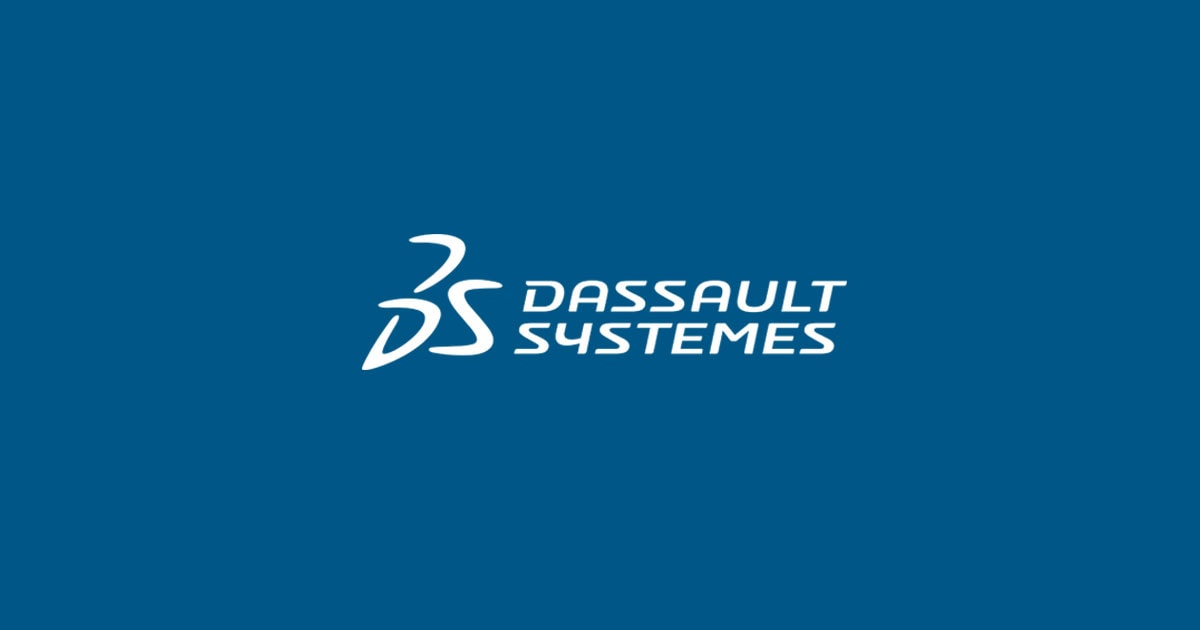February 15, 2019

# STATISTICAL VALIDATION with GEMS

The importance of the Validation of the Block Model Estimation with GEOVIA…Maria Angelica Gonzalez

The importance of the Validation of the Block Model Estimation with GEOVIA GEMS: STATISTICAL VALIDATION.

When estimating a block model, no matter what interpolation method is chosen, it is essential to validate the obtained results in order to ensure the estimation process quality according to the current industry standards.

By validating the block model, we look for:

• That the estimation be globally unbiased
• Minimize local bias
• Keep the estimation smoothing at a reasonably acceptable level

There are numerous methods to do this and through upcoming blogs we will discuss these three (3):

1. Graphical validation.
2. Statistical validation.
3. Swath Plot validation.

Next, we will explain the method of Statistical Validation:

STATISTICAL VALIDATION OF THE BLOCK MODEL ESTIMATION

Objective: Validate that the estimation is globally unbiased, that is to say, that the global mean of the estimation (blocks) is the same as the global mean of the “real” data (declustered composites).

How to do it? Basic statistics are reported by domain for the estimated blocks and for the declustered composites; the global bias is calculated, as follows:

Stay up to date